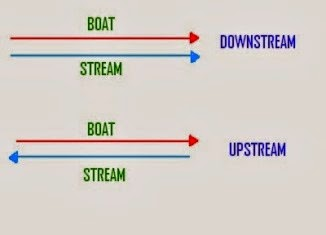Boats and Stream Section
u – Speed of boat/man
v – Speed of water
Speed of boat along the river (Downstream) a = u + v
Speed of boat against the river (Upstream) b = u – v
Mostly speed if boat greater than speed of water
Speed of boat in still water u = ½(a + b)
Speed of water current v = ½(a – b)
Distance = time (u2 - v2)/ 2u

1) A man can row 18km/hr in still water. speed of the man in downstream is thrice the speed in upstream. Find the rate of stream.
Solution:
Let, Speed of man in upstream a = a
Speed of man in downstream b = 3a
Speed of man in still water u = ½(a + b)
Speed of man in still water = ½(3a + a) = 2a
We know speed of man in still water = 18
So, a = 9
Rate of stream = ½(27 – 9) =9 km/hr
2) A boat can cover certain distance in downstream in 1hr. and it takes 1½hr to cover same distance in upstream. If speed of the stream is 3kmph, then what will be the speed of boat?
Solution:
let speed of boat in still water be x
speed in downstream = x + 3
speed in upstream = x – 3
Since boat covered same distance in upstream and downstream,
(x + 3)*1 = (x – 3)*(3/2)
speed in downstream x= 15 kmph
3) A man can row three quarter of a km against the stream in 11¼ min and down the stream in 7½min. what is the speed of man in still water.
Solution:
Speed of man in Upstream = ((¾)/(45/4)) *60
= 4 kmph
Speed of man in Downstream = ((¾)/(15/4))* 60
= 12 kmph
Speed of man in still water u = ½(a + b)
Speed of man in still water = (12 + 4)*½ = 8 kmph
4) A streamer takes 3hr to cover a distance of 24km upstream, if the rate of stream is 3 kmph. Then find the speed of streamer in still water.
Solution:
Upstream speed = 24/3 =8kmph
Rate of stream = 3kmph
Speed of streamer – rate of stream = upstream
Speed of streamer = 11kmph
5) The distance between two points is 36km. A boat rows in still water at 6kmph, it takes 8hr less to cover dist in downstream in comparison to that in upstream. Find the rate of stream.
Solution:
Time = distance * speed
Difference between time taken to cover upstream and downstream is 8hr
(36/(6-x)) – (36/(6+x)) = 8
36(6+x) – 36(6-x) = 8(36-x2)
9x = 36- x2
x2+9x-36 = 0 (to find value of x use quadratic equation technique)
(x+12)(x-3)=0
Speed cannot be negative so x = 3kmph
Rate of stream = 3kmph

Source:- affairscloud
Mathematics Booster for Boats and Stream Section for SSC,Bank examsReviewed by Newstechcafe on 00:33:00 Rating: 5

#### 1 comment:

1.This Post Will Help Students Preparing for Aptitude in NON GATE PSUs